# My Puzzle for the Day

In November 2018 the BBC Radio 4 Today Programme was visiting Ulster University for an outside broadcast. I was asked to write the Puzzle for the Day for the broadcast. Here is my puzzle and some discussion about how it can be solved. The puzzle and a very brief solution is on the BBC page, but I restate it below, together with a more full solution.

Part One

A Professor gives her team of three PhD students many calculations to perform. The first student is the most experienced and can complete the job on her own in 7 hours, the next would take 14 hours on his own, and the last would take 28 hours working single handed to complete the task. How long would the task take if they all worked together?

Part Two

If the Professor has more helpers, but which follow the same pattern of numbers to complete the task, what is the absolute minimum time the task can take?

### Solving Part One.

Possibly the easiest way to solve the first part is to consider how much work can be done by each team member in a single hour and adding to get the total amount of work done per hour. For instance, the first team member can do the whole job in seven hours, so they can doof the job in one hour etc. So the team together can do the following in one hour:In other words, one quarter of the job can be done in a single hour by the team of three working together. It follows that to do the whole job, the team needs four hours.

### Solving Part Two.

So what about part two of the puzzle? Quite reasonably, some people attempting the puzzle assumed that the more and more team members we would add, the time to complete the task would eventually drop to zero. This seems fairly intuitive – if you have potentially infinite team members then the total time must drop to zero.

But imagine we continue our pattern from above, for far more than three team members. This would be the proportion of work done in one hour by even an infinite team.If this addition produces an infinite “answer” then an infinite work rate per hour would certainly suggest the task could be done instantly. Surprisingly perhaps this sum of infinitely many numbers does not have an infinite answer. It may be a little easier to see its nature if we take out a factor of.

Then the summation looks like this: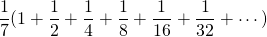It may be intuitive for some people that the numbers inside the bracket will get over closer to 2 – setting aside the initial 1, each subsequent number added goes half way from the previous sum to adding an additional 1 in total. In other words, this whole sum will be.

Some infinite summations do indeed have finite answers, but it can be quite difficult to prove which are which, or to find the summations if they are finite. However, this example, aside from intuitively having a relatively easy answer falls into a special category of such summations called a geometric progression. These are series of the form: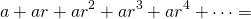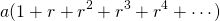In other words, each item in the sum is the previous item multiplied by some common ratio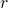. There is a nice formula for the sum of the first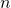terms of such progressions which you could use to solve part one – though it would rather be a sledgehammer to crack a nutshell, but there is a formula for the infinite summation too.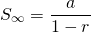(provided, or in other words thatis between -1 and 1 not-inclusive, if not then the summation is infinite).

In this case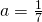and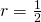andpasses the test above so the infinite sum is indeed verified to be.

Finally therefore, if even infinitely many team members can only doof the job in an hour, we need to work out the number to multiply on this to get to the whole job being done, i.e. not “2/7” of the job but the whole “1” of the job.

That number is, so the whole job can be reduced from the four hours of part one to not less than 3.5 hours.

Of course, there are other ways to solve the puzzle. This is just one example pathway. If you are interested in how I went about constructing the puzzle, I detail that in another post.

Incidentally, the fact that summations of infinitely many objects sometimes has finite answers is of vital importance for many real life applications of mathematics. The whole subject of Integral Calculus relies on this, and for instance, this is closely related to why it is possible, with finite energy for a rocket to escape Earth or an electron to escape an atom.